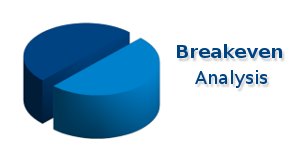Categories

# Breakeven CalculationLet’s say you’re trying to decide whether or not to produce a certain product. Say a new type of cuddly teddy bear. You’ll inevitably ask yourself “how many teddy bears do I need to sell to break even?” Breakeven analysis helps you answer that question.

The first step is to figure out the costs involved. This is the toughest part because there are two types of costs – fixed costs and variable costs. You need to figure out both.

Fixed costs: These are costs that you have to pay regardless of how many units you produce. In our teddy bear example, fixed costs would include:

• the cost you pay an artist to sketch out the bear so a prototype can be made
• the cost of a prototype
• what it costs you in time (and/or gas money) to find an appropriate manufacturer
• and so on…

The point is that you’d have to pay these costs even if you only produced one teddy bear.

Variable costs: These are the costs for each additional unit produced. Continuing with our teddy bear example, each bear is made up of materials (such as fabric, foam stuffing etc.) that you have to pay for. The more bears you produce, the more you have to pay in materials. These materials are variable costs because they vary with the number of units produced.

Total Cost: Once you’ve figured out your fixed costs and your variable costs, you can get your total cost. The total cost will also depend on how many units you decide to produce. It’s pretty obvious that the more bears you make, the more it will cost you. Let’s say we calculated our fixed costs to total \$5000. As for variable costs, we determined that it will cost \$5 to produce each additional teddy bear.

For example, let’s say we decide to produce 2000 bears:

Total cost = fixed costs + (variable costs × quantity produced)
Total cost = \$5000 + (\$5 × 2000)
Total cost = \$5000 + \$10,000

Therefore, the cost to produce 100 units is:

Total cost = \$15,000

Price: The final ingredient to find your breakeven point is to the price you’re going to sell the teddy bears for. Let’s say you determine the optimal price is \$35.00. (For tips on setting a price, see our pricing article: Setting a price) In our example, this means that each bear will contribute \$30.00 (\$35.00 – \$5.00) to covering your fixed costs and earning profit.

As a side note, accounting nerds call the difference between the price and the variable cost per unit the contribution margin per unit. As shown above, the contribution margin for our example is \$30.

Finally, we show how to get the breakeven point:

Profit = Revenues – Costs
Profit = (Price x Quantity) – (Fixed Costs + (Variable Costs × Quantity))

The breakeven point means that profit is \$0 by definition. So we can use \$0 for profit in our formula above.

0 = (Price x Quantity) – (Fixed Costs + (Variable Costs × Quantity))

Rearranging the above formula we can show that the quantity at which profit is \$0 is:

Quantity = Fixed Costs ÷ (Price – Variable Costs)

For this teddy bear example the number of units we need to sell to breakeven is:

Breakeven point in units = \$5000 ÷ (\$35 – \$5)
Breakeven point in units = \$5000 ÷ \$30
Breakeven point in units = 167

Therefore you would need to sell 167 teddy bears to cover all of your costs.

## Make Life Easy With a Breakeven Calculator

It’s beneficial to use understand the principles outlined above, but why not make life easier? Instead of bothering with the formulas above, use our free breakeven and profitability calculator to save time.